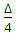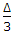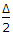# Civil Engineering - Surveying

Exercise : Surveying - Section 3
41.
If α is the angle between the polar ray and the tangent at the point of commencement of a lemniscate curve, the equation of the curve, is
l = ksin α
l = ksin 2α
l = ksin 3α
l = ktan 2α
l = kcos 2α
Explanation:
No answer description is available. Let's discuss.

42.
Pick up the correct statement from the following :
If the slope of the curve of a mass diagram in the direction of increasing abscissa is downward, it indicates an embankment
The vertical distance between a maximum ordinate and the next forward maximum ordinate represents the whole volume of the embankment
The vertical distance between a minimum ordinate and the next forward maximum ordinate represents the whole volume of a cutting
The area enclosed by a loop of the curve and balancing line, measures the haul in that direction.
all the above.
Explanation:
No answer description is available. Let's discuss.

43.
Pick up the correct statement from the following :
if the image of the object does not fall on the plane of the cross-lines, parallax exists
parallax has nothing to do with the eyepiece
the eyepiece is adjusted for clear vision of the cross hairs
all the above.
Explanation:
No answer description is available. Let's discuss.

44.
The slope correction may be ignored if
the slope of the ground is less than 3°
to slope of the ground is say 1 in 19
both (a) and (b)
neither (a) nor (b)